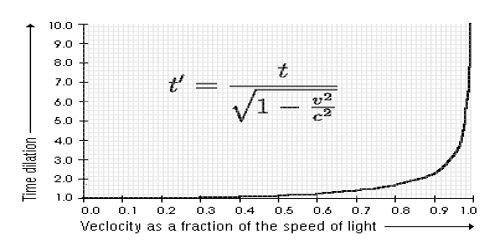Physics

# Time DilationTime Dilation: A clock in a moving frame will measure slower time compared to that in a clock in a stationary frame of reference i.e., time in this frame will increase. This phenomenon is called time dilation.

It is a slowing of time in accordance with the theory of relativity that occurs in a system in motion relative to an outside observer and that becomes perceptible particularly as the speed of the system approaches that of light. Time dilation and length contraction phenomena happen in the journey to space. Due to travel to a long distance, even to make the journey to our nearest planet it takes a long time.

The equation for calculating time dilation is as follows: t = t0/(1-v2/c2)1/2

where; t = time observed in the other reference frame

t0 = time in observers own frame of reference (rest time)

v = the speed of the moving object

c = the speed of light in a vacuum

so in our problem we will let v = .95c, t0 = 10 years and we will solve for t which is the time that the earthbound brother measures.

t = 10/(1- (.95c)2/c2)1/2

t = 10/(1- .952)1/2

t = 10/ .312

t = 32 years (the time the earthbound brother measures)